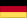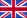Findings
of the static analysis of the arch dam Karakaya in Turkey
by Dr. sc. techn. ETH Rudolf Trinkner

# 7. Comparison of the Conclusions with those of the Difference Method and Model Test

Appendix, Images 9 through 13 are selected graphs comparing the stresses and displacements determined via the finite element method, the difference method (final design), and the model test. Of particular importance is the observation that results of the finite element calculation generally lies between those of the other two methods. The model test provides the largest values for stresses and displacements. The displacements were split into radial and tangential directions to allow comparisons with earlier analyses.

The graphs in Appendix Image 9 show the 'vertical' stresses in the centre of spillway for hy-drostatic loads only. Due to the predominant shell action in this zone, compressive stresses prevail on the water side surface of the dam whereas tensile stresses prevail on the air side of the dam. On the basis of the model tests, it seems that the results of the finite element analysis are closer to reality than those of the difference method (final design).

The graph shows comparatively high tensile stresses on the water side surface below 550 m.a.s.l. The resulting tensile stresses of superimposition of the load cases self-weight and hy-drostatic pressure however never exceed a value 18 kp/cm2.

Appendix, Image 10 shows the graph of the horizontal stresses in the centre of the spillway. The graph shows that the horizontal stresses reduce with decreasing elevation. From this it can be derived that the arching effect prevails in the upper sections whereas console force prevails in the lower sections.

Appendix, Image 11 shows the horizontal stresses at elevation of 675 m.a.s.l. The significant discontinuities at about 90 m left and right of the dam centre are noteworthy. This irregularity relates to the stress concentration at points with significant changes to the outline of the dam at the sides of the spillway.

Appendix, Images 12 and 13 show the graphs of the radial and tangential displacements of the shear surface of the dam in the centre of the spillway and at elevation of 675 m.a.s.l.

Die radialen Verschiebungen, ermittelt mit der Finite Elementmethode, sind nur wenig größer als jene, welche mit dem Differenzenverfahren (final Design) gewonnen wurden. Dies kann damit in Zusammenhang gebracht werden, dass bei der Differenzenrechnung

The radial displacements as calculated using the finite element method are slightly larger than those calculated using the difference method (final design). This is directly linked to the fact that shear deformations are neglected using the difference method (theory of thin shells). Whereas the finite element method includes these additional deformations (hybrid expres-sion).

It can be observed, that the radial displacements measured on the model are significantly lar-ger than those calculated. This phenomenon can be related to the following assumptions for the analytical methods:

• The dam is based on the plane surface of the elastic half-space (Vogt elements).
• The elastic moduli of the dam body as well as those of the rock abutments were assumed to be constant during loading and unloading (that is the dam concrete and the surrounding rock possess an ideal elasticity; there is no hysteresis).

In reality, these theoretical assumptions present additional links, which cannot be reproduced on the model. Which means that the model will always produce larger deformations.